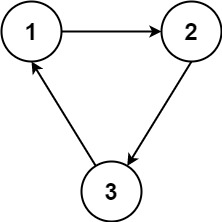1136. Parallel Courses

You are given an integer `n` which indicates that we have `n` courses, labeled from `1` to `n`. You are also given an array `relations` where `relations[i] = [a, b]`, representing a prerequisite relationship between course `a` and course `b`: course `a` has to be studied before course `b`.

In one semester, you can study any number of courses as long as you have studied all the prerequisites for the course you are studying.

Return the minimum number of semesters needed to study all courses. If there is no way to study all the courses, return `-1`.

Example 1:```Input: n = 3, relations = [[1,3],[2,3]]
Output: 2
Explanation: In the first semester, courses 1 and 2 are studied. In the second semester, course 3 is studied.
```

Example 2:```Input: n = 3, relations = [[1,2],[2,3],[3,1]]
Output: -1
Explanation: No course can be studied because they depend on each other.
```

Constraints:

• `1 <= n <= 5000`
• `1 <= relations.length <= 5000`
• `1 <= a, b <= n`
• `a != b`
• All the pairs `[a, b]` are unique.

1136. Parallel Courses
``````struct Solution;
use std::collections::VecDeque;

impl Solution {
fn minimum_semesters(n: i32, relations: Vec<Vec<i32>>) -> i32 {
let n = n as usize;
let mut adj = vec![vec![]; n];
let mut degree = vec![0; n];
for edge in relations {
let x = edge as usize - 1;
let y = edge as usize - 1;
degree[y] += 1;
}
let mut visited = vec![false; n];
let mut queue = VecDeque::new();
for i in 0..n {
if degree[i] == 0 {
visited[i] = true;
queue.push_back(i);
}
}
let mut res = 0;
while !queue.is_empty() {
let n = queue.len();
res += 1;
for _ in 0..n {
let u = queue.pop_front().unwrap();
degree[v] -= 1;
if !visited[v] && degree[v] == 0 {
visited[v] = true;
queue.push_back(v);
}
}
}
}
if visited.into_iter().all(|x| x) {
res
} else {
-1
}
}
}

#[test]
fn test() {
let n = 3;
let relations = vec_vec_i32![[1, 3], [2, 3]];
let res = 2;
assert_eq!(Solution::minimum_semesters(n, relations), res);
let n = 3;
let relations = vec_vec_i32![[1, 2], [2, 3], [3, 1]];
let res = -1;
assert_eq!(Solution::minimum_semesters(n, relations), res);
}
``````